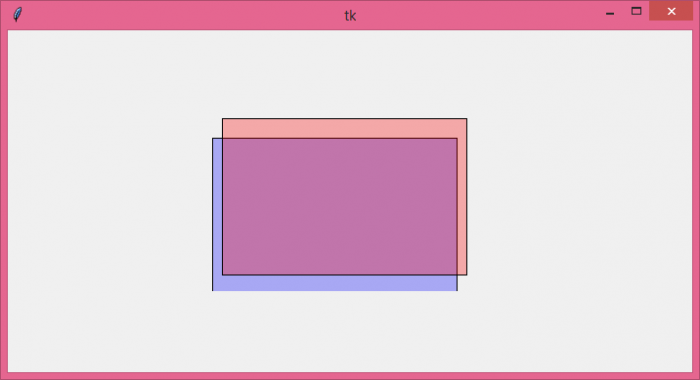# How can I vary a shape's alpha with Tkinter?

The Canvas widget is one of the multilateral widgets in tkinter library which is used to provide graphics for any application. It can be used to draw shapes, images, animating objects or any complex visuals. The alpha property of the shape defines that if we give the alpha value to any shape, then it must have some transparency behaviour with respect to its parent window.

To define the alpha property, we have to assume that every shape have some colors in it and whenever we provide an alpha value to a shape, then it must be converted into an Image. The Image can be displayed using the Canvas widget.

## Example

# Import the required libraries
from tkinter import *
from PIL import Image, ImageTk

# Create an instance of tkinter frame
win= Tk()

# Set the size of the tkinter window
win.geometry("700x350")

# Store newly created image
images=[]

# Define a function to make the transparent rectangle
def create_rectangle(x,y,a,b,**options):
if 'alpha' in options:
# Calculate the alpha transparency for every color(RGB)
alpha = int(options.pop('alpha') * 255)
# Use the fill variable to fill the shape with transparent color
fill = options.pop('fill')
fill = win.winfo_rgb(fill) + (alpha,)
image = Image.new('RGBA', (a-x, b-y), fill)
images.append(ImageTk.PhotoImage(image))
canvas.create_image(x, y, image=images[-1], anchor='nw')
canvas.create_rectangle(x, y,a,b, **options)
canvas= Canvas(win)

# Create a rectangle in canvas
create_rectangle(50, 110,300,280, fill= "blue", alpha=.3)
create_rectangle(60, 90,310,250, fill= "red", alpha=.3)

canvas.pack()

win.mainloop()

## Output

Run the above code to see how the alpha property varies in shapes.